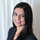# Showing a Confidence Interval - R and Periscope Visualization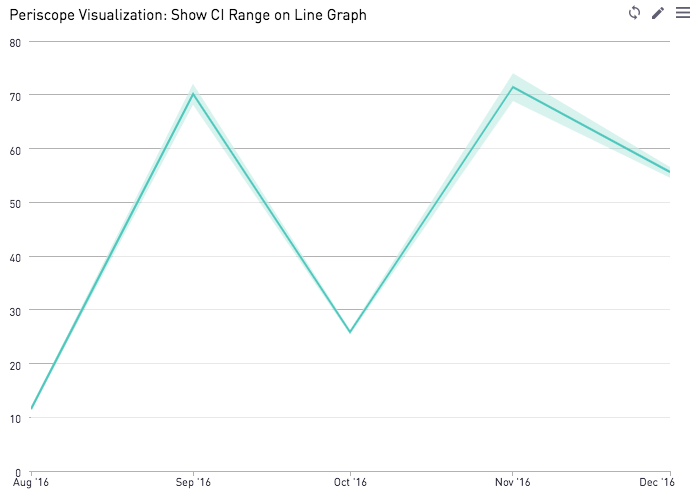Confidence intervals are a favorite among many professionals with a statistics background. We can use the R integration in Periscope to show the Confidence interval as a shaded region around a line of means from period to period, as shown in the image below.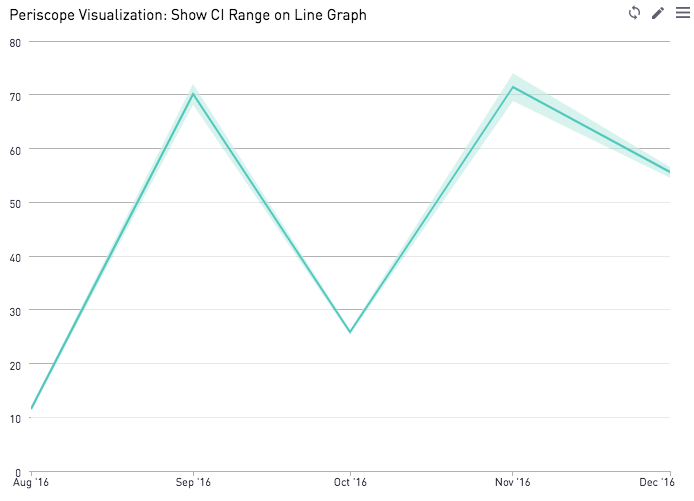How to Interpret a confidence interval: if we computed the X% confidence interval for multiple samples, X% of the samples will contain the true population mean. The most common confidence interval used is 95%. However other confidence intervals such as 90% and 99% also are utilized in certain applications. Here's a helpful resource if you want to learn more about Confidence Intervals and the mathematics behind it.

Looking for a single confidence interval printout instead? Check out our post here!

In this example, the SQL output is a data frame that contains data about the amount of money spent per user per month on a fictional gaming app. The 3 columns of this dataset are:

• User_id
• My_month
• Val (the total amount of money spent)

Below is the R snippet used to generate the final data frame that forms the basis of the above visualization. Note, this calculates the confidence interval using the Z test statistic (so ensure your sample is normally distributed) and uses unpaired means (we assume that each sample comprises of different individuals)

```# SQL output is imported as a dataframe variable called "df"
# Use Periscope to visualize a dataframe or show text by passing data to periscope.table() or periscope.text() respectively. Show an image by calling periscope.image() after your plot.
library(dplyr)

CIrange <- function(df, alpha = 0.95){
z = qnorm((1 - alpha)/2)
df <- df %>%
group_by(my_month) %>%
summarise_all(funs(mean, sd, n()))
df\$CIwidth = 2*z * df\$val_sd / sqrt(df\$val_n)
df\$lower_bound <- df\$val_mean - z * df\$val_sd / sqrt(df\$val_n)
return(df)
}

periscope.table(CIrange(df))```

Notice how the default value for the CIrange function is 95%, but other CI ranges can easily be set by fixing this parameter to another value.

In the visualization settings, we set my_month as the x axis. Val_mean, CIwidth, and lower_bound are all Y values.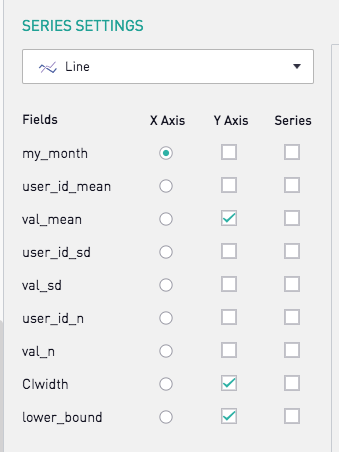Next, we scroll down to set the series type for CIwidth and lower_bound as Area. We shade the lower_bound series white to give the illusion of a nice CI range that frames the mean average line. Stylistically, I like setting the CIwidth to be a lighter shade of the mean average line color.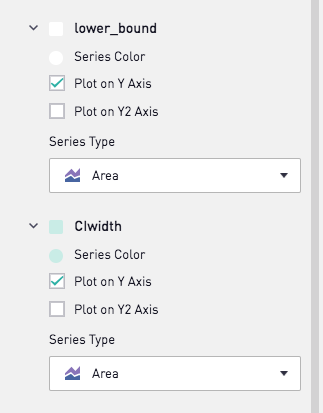Want to see more visualization tips like this? Comment below!